College Algebra with Corequisite Support 2e

# 7.2Systems of Linear Equations: Three Variables

College Algebra with Corequisite Support 2e7.2 Systems of Linear Equations: Three Variables

### Learning Objectives

In this section, you will:

• Solve systems of three equations in three variables.
• Identify inconsistent systems of equations containing three variables.
• Express the solution of a system of dependent equations containing three variables.

### Corequisite Skills

#### Learning Objectives

• Determine whether an ordered triple is a solution of a system of three linear equations with three variables (IA 4.4.1)
• Solve a system of three linear equations with three variables (IA 4.4.2)

#### Objective 1: Determine whether an ordered triple is a solution of a system of three linear equations with three variables (IA 4.4.1)

A linear equation with three variables where a, b, c, and d are real numbers and a, b, and c are not all 0, is of the form $ax+by+cz=dax+by+cz=d$ . The graph of a linear equation with three variables is a plane.

A system of linear equations with three variables is a set of linear equations with three variables. For example,

$3x+y+z=2x+2y+z=-33x+y+2z=43x+y+z=2x+2y+z=-33x+y+2z=4$

is a system of linear equations with three variables.

Solutions of a system of equations are the values of the variables that make all the equations true. A solution is represented by an ordered triple (x,y,z).

### Example 1

Determine whether the ordered triples are solutions to the given system.

$3x+y+z=2x+2y+z=-33x+y+2z=43x+y+z=2x+2y+z=-33x+y+2z=4$ at $(1, -3, 2)(1, -3, 2)$ and at $(4, -1, -5)(4, -1, -5)$

##### Practice Makes Perfect

Determine whether the ordered pairs are solutions to the given system.

$2x–6y+z=3 3x–4y–3z=2at(3,1,3)and at(4,3,7)2x+y–2z=3 2x–6y+z=3 3x–4y–3z=2at(3,1,3)and at(4,3,7)2x+y–2z=3$
1.

At (3, 1, 3):

 $2x–6y+z=32x–6y+z=3$ $3x–4y–3z=23x–4y–3z=2$ $2x+3y–2z=32x+3y–2z=3$ ________________________ ________________________ ________________________ Conclusion: ________________________
2.

At (4, 3, 7):

 $2x–6y+z=32x–6y+z=3$ $3x–4y–3z=23x–4y–3z=2$ $2x+3y–2z=32x+3y–2z=3$ ________________________ ________________________ ________________________ Conclusion: ________________________

#### Objective 2: Solve a system of three linear equations with three variables (IA 4.4.2)

When we solve a system of linear equations with three variables, we have many possible solutions.

The solutions are summarized in the table below.

 One Solution The three planes intersect at a common point.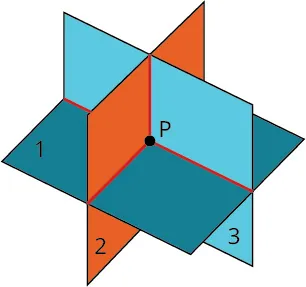No Solution The planes are parallel; they have no points in common.Two planes are the same and they are parallel to the third plane. They have no points in common.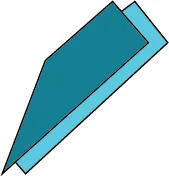Two planes are parallel and they each intersect the third plane. They have no points in common.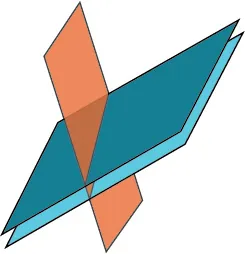Each plane intersects the other two but they have no points in common.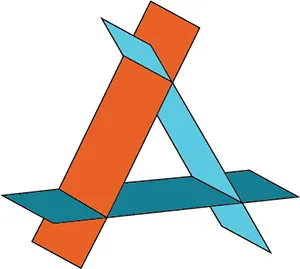Infinitely many solutions The three planes intersect in one line. They have many points on that line in common.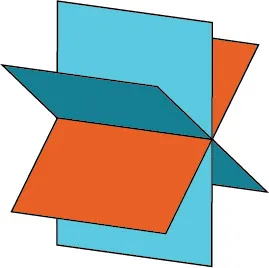Two planes are the same and intersect the third one in a line. they have many points on that line in common.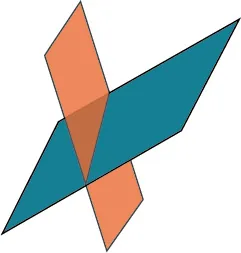The three planes are exactly the same. They have many points in common.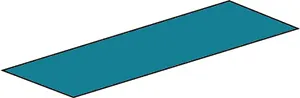### How To

#### Solve a system of linear equations with three variables.

1. Step 1. Write the equations in standard form. If any coefficients are fractions, clear them.
2. Step 2.
Eliminate the same variable from two equations.
1. Decide which variable you will eliminate.
2. Work with a pair of equations to eliminate the chosen variable.
3. Multiply one or both equations so that the coefficients of that variable are opposites.
4. Add the equations resulting from Step 2 to eliminate one variable.
3. Step 3. Repeat Step 2 using two other equations and eliminate the same variable as in Step 2.
4. Step 4. The two new equations form a system of two equations with two variables. Solve this system.
5. Step 5. Use the values of the two variables found in Step 4 to find the third variable.
6. Step 6. Write the solution as an ordered triple.
7. Step 7. Check that the ordered triple is a solution to all three original equations

### Example 2

Solve the system of equations: ${x+2y−z=12x+7y+4z=11x+3y+z=4.{x+2y−z=12x+7y+4z=11x+3y+z=4.$

### Try It #1

Solve the system of linear equations with three variables.

$x+2y-3z=-1 x-3y+z=12x-y-2z=2(1)(2)(3)x+2y-3z=-1 x-3y+z=12x-y-2z=2(1)(2)(3)$
 Step 1 Write the equations in standard form. If any coefficients are fractions, clear them.________________________________________ Step 2 Let's use equations (1) and (2) to eliminate $xx$________________________________________ Step 3 Let's use equations (1) and (3) to eliminate $xx$________________________________________ Step 4 Let's now use the new equations (4) and (5) to eliminate $yy$________________________________________ Step 5 Step 6
##### Practice Makes Perfect
3.

Determine whether the ordered pair is a solution to the given system
$y-10z=-82x-y=2x-5z=3at(7,12,2)and at(2,2,1)y-10z=-82x-y=2x-5z=3at(7,12,2)and at(2,2,1)$

Figure 1 (credit: “Elembis,” Wikimedia Commons)

### Try It #2

Solve the system of equations in three variables.

$2x+y−2z=−1 3x−3y−z=5 x−2y+3z=6 2x+y−2z=−1 3x−3y−z=5 x−2y+3z=6$

### Identifying Inconsistent Systems of Equations Containing Three Variables

Just as with systems of equations in two variables, we may come across an inconsistent system of equations in three variables, which means that it does not have a solution that satisfies all three equations. The equations could represent three parallel planes, two parallel planes and one intersecting plane, or three planes that intersect the other two but not at the same location. The process of elimination will result in a false statement, such as $3=7 3=7$ or some other contradiction.

### Example 4

#### Solving an Inconsistent System of Three Equations in Three Variables

Solve the following system.

$x−3y+z=4 (1) −x+2y−5z=3 (2) 5x−13y+13z=8 (3) x−3y+z=4 (1) −x+2y−5z=3 (2) 5x−13y+13z=8 (3)$

#### Analysis

In this system, each plane intersects the other two, but not at the same location. Therefore, the system is inconsistent.

### Try It #3

Solve the system of three equations in three variables.

$x+y+z=2 y−3z=1 2x+y+5z=0 x+y+z=2 y−3z=1 2x+y+5z=0$

### Expressing the Solution of a System of Dependent Equations Containing Three Variables

We know from working with systems of equations in two variables that a dependent system of equations has an infinite number of solutions. The same is true for dependent systems of equations in three variables. An infinite number of solutions can result from several situations. The three planes could be the same, so that a solution to one equation will be the solution to the other two equations. All three equations could be different but they intersect on a line, which has infinite solutions. Or two of the equations could be the same and intersect the third on a line.

### Example 5

#### Finding the Solution to a Dependent System of Equations

Find the solution to the given system of three equations in three variables.

$2x+y−3z=0 (1) 4x+2y−6z=0 (2) x−y+z=0 (3) 2x+y−3z=0 (1) 4x+2y−6z=0 (2) x−y+z=0 (3)$

#### Analysis

As shown in Figure 5, two of the planes are the same and they intersect the third plane on a line. The solution set is infinite, as all points along the intersection line will satisfy all three equations.

Figure 5

### Q&A

Does the generic solution to a dependent system always have to be written in terms of $x? x?$

No, you can write the generic solution in terms of any of the variables, but it is common to write it in terms of x and if needed $x x$ and $y. y.$

### Try It #4

Solve the following system.

$x+y+z=7 3x−2y−z=4 x+6y+5z=24 x+y+z=7 3x−2y−z=4 x+6y+5z=24$

### Media

Access these online resources for additional instruction and practice with systems of equations in three variables.

### 7.2 Section Exercises

#### Verbal

1.

Can a linear system of three equations have exactly two solutions? Explain why or why not

2.

If a given ordered triple solves the system of equations, is that solution unique? If so, explain why. If not, give an example where it is not unique.

3.

If a given ordered triple does not solve the system of equations, is there no solution? If so, explain why. If not, give an example.

4.

Using the method of addition, is there only one way to solve the system?

5.

Can you explain whether there can be only one method to solve a linear system of equations? If yes, give an example of such a system of equations. If not, explain why not.

#### Algebraic

For the following exercises, determine whether the ordered triple given is the solution to the system of equations.

6.

$2x−6y+6z=−12 x+4y+5z=−1 −x+2y+3z=−1 2x−6y+6z=−12 x+4y+5z=−1 −x+2y+3z=−1$ and $(0,1,−1) (0,1,−1)$

7.

$6x−y+3z=6 3x+5y+2z=0 x+y=0 6x−y+3z=6 3x+5y+2z=0 x+y=0$ and $(3,−3,−5) (3,−3,−5)$

8.

$6x−7y+z=2 −x−y+3z=4 2x+y−z=1 6x−7y+z=2 −x−y+3z=4 2x+y−z=1$ and $(4,2,−6) (4,2,−6)$

9.

$x−y=0 x−z=5 x−y+z=−1 x−y=0 x−z=5 x−y+z=−1$ and $(4,4,−1) (4,4,−1)$

10.

$-x−y+2z=3 5x+8y−3z=4 -x+3y−5z=−5 -x−y+2z=3 5x+8y−3z=4 -x+3y−5z=−5$ and $(4,1,−7) (4,1,−7)$

For the following exercises, solve each system by elimination.

11.

$3x−4y+2z=−15 2x+4y+z=16 2x+3y+5z=20 3x−4y+2z=−15 2x+4y+z=16 2x+3y+5z=20$

12.

$5x−2y+3z=20 2x−4y−3z=−9 x+6y−8z=21 5x−2y+3z=20 2x−4y−3z=−9 x+6y−8z=21$

13.

$5x+2y+4z=9 −3x+2y+z=10 4x−3y+5z=−3 5x+2y+4z=9 −3x+2y+z=10 4x−3y+5z=−3$

14.

$4x−3y+5z=31 −x+2y+4z=20 x+5y−2z=−29 4x−3y+5z=31 −x+2y+4z=20 x+5y−2z=−29$

15.

$5x−2y+3z=4 −4x+6y−7z=−1 3x+2y−z=4 5x−2y+3z=4 −4x+6y−7z=−1 3x+2y−z=4$

16.

$4x+6y+9z=0 −5x+2y−6z=3 7x−4y+3z=−3 4x+6y+9z=0 −5x+2y−6z=3 7x−4y+3z=−3$

For the following exercises, solve each system by Gaussian elimination.

17.

$2x−y+3z=17 −5x+4y−2z=−46 2y+5z=−7 2x−y+3z=17 −5x+4y−2z=−46 2y+5z=−7$

18.

$5x−6y+3z=50 −x+4y=10 2x−z=10 5x−6y+3z=50 −x+4y=10 2x−z=10$

19.

$2x+3y−6z=1 −4x−6y+12z=−2 x+2y+5z=10 2x+3y−6z=1 −4x−6y+12z=−2 x+2y+5z=10$

20.

$4x+6y−2z=8 6x+9y−3z=12 −2x−3y+z=−4 4x+6y−2z=8 6x+9y−3z=12 −2x−3y+z=−4$

21.

$2x+3y−4z=5 −3x+2y+z=11 −x+5y+3z=4 2x+3y−4z=5 −3x+2y+z=11 −x+5y+3z=4$

22.

$10x+2y−14z=8 −x−2y−4z=−1 −12x−6y+6z=−12 10x+2y−14z=8 −x−2y−4z=−1 −12x−6y+6z=−12$

23.

$x+y+z=14 2y+3z=−14 −16y−24z=−112 x+y+z=14 2y+3z=−14 −16y−24z=−112$

24.

$5x−3y+4z=−1 −4x+2y−3z=0 −x+5y+7z=−11 5x−3y+4z=−1 −4x+2y−3z=0 −x+5y+7z=−11$

25.

$x+y+z=0 2x−y+3z=0 x−z=0 x+y+z=0 2x−y+3z=0 x−z=0$

26.

$3x+2y−5z=6 5x−4y+3z=−12 4x+5y−2z=15 3x+2y−5z=6 5x−4y+3z=−12 4x+5y−2z=15$

27.

$x+y+z=0 2x−y+3z=0 x−z=1 x+y+z=0 2x−y+3z=0 x−z=1$

28.

$3x− 1 2 y−z=− 1 2 4x+z=3 −x+ 3 2 y= 5 2 3x− 1 2 y−z=− 1 2 4x+z=3 −x+ 3 2 y= 5 2$

29.

$6x−5y+6z=38 1 5 x− 1 2 y+ 3 5 z=1 −4x− 3 2 y−z=−74 6x−5y+6z=38 1 5 x− 1 2 y+ 3 5 z=1 −4x− 3 2 y−z=−74$

30.

$1 2 x− 1 5 y+ 2 5 z=− 13 10 1 4 x− 2 5 y− 1 5 z=− 7 20 − 1 2 x− 3 4 y− 1 2 z=− 5 4 1 2 x− 1 5 y+ 2 5 z=− 13 10 1 4 x− 2 5 y− 1 5 z=− 7 20 − 1 2 x− 3 4 y− 1 2 z=− 5 4$

31.

$− 1 3 x− 1 2 y− 1 4 z= 3 4 − 1 2 x− 1 4 y− 1 2 z=2 − 1 4 x− 3 4 y− 1 2 z=− 1 2 − 1 3 x− 1 2 y− 1 4 z= 3 4 − 1 2 x− 1 4 y− 1 2 z=2 − 1 4 x− 3 4 y− 1 2 z=− 1 2$

32.

$1 2 x− 1 4 y+ 3 4 z=0 1 4 x− 1 10 y+ 2 5 z=−2 1 8 x+ 1 5 y− 1 8 z=2 1 2 x− 1 4 y+ 3 4 z=0 1 4 x− 1 10 y+ 2 5 z=−2 1 8 x+ 1 5 y− 1 8 z=2$

33.

$4 5 x− 7 8 y+ 1 2 z=1 − 4 5 x− 3 4 y+ 1 3 z=−8 − 2 5 x− 7 8 y+ 1 2 z=−5 4 5 x− 7 8 y+ 1 2 z=1 − 4 5 x− 3 4 y+ 1 3 z=−8 − 2 5 x− 7 8 y+ 1 2 z=−5$

34.

$− 1 3 x− 1 8 y+ 1 6 z=− 4 3 − 2 3 x− 7 8 y+ 1 3 z=− 23 3 − 1 3 x− 5 8 y+ 5 6 z=0 − 1 3 x− 1 8 y+ 1 6 z=− 4 3 − 2 3 x− 7 8 y+ 1 3 z=− 23 3 − 1 3 x− 5 8 y+ 5 6 z=0$

35.

$− 1 4 x− 5 4 y+ 5 2 z=−5 − 1 2 x− 5 3 y+ 5 4 z= 55 12 − 1 3 x− 1 3 y+ 1 3 z= 5 3 − 1 4 x− 5 4 y+ 5 2 z=−5 − 1 2 x− 5 3 y+ 5 4 z= 55 12 − 1 3 x− 1 3 y+ 1 3 z= 5 3$

36.

$1 40 x+ 1 60 y+ 1 80 z= 1 100 − 1 2 x− 1 3 y− 1 4 z=− 1 5 3 8 x+ 3 12 y+ 3 16 z= 3 20 1 40 x+ 1 60 y+ 1 80 z= 1 100 − 1 2 x− 1 3 y− 1 4 z=− 1 5 3 8 x+ 3 12 y+ 3 16 z= 3 20$

37.

$0.1x−0.2y+0.3z=2 0.5x−0.1y+0.4z=8 0.7x−0.2y+0.3z=8 0.1x−0.2y+0.3z=2 0.5x−0.1y+0.4z=8 0.7x−0.2y+0.3z=8$

38.

$0.2x+0.1y−0.3z=0.2 0.8x+0.4y−1.2z=0.1 1.6x+0.8y−2.4z=0.2 0.2x+0.1y−0.3z=0.2 0.8x+0.4y−1.2z=0.1 1.6x+0.8y−2.4z=0.2$

39.

$1.1x+0.7y−3.1z=−1.79 2.1x+0.5y−1.6z=−0.13 0.5x+0.4y−0.5z=−0.07 1.1x+0.7y−3.1z=−1.79 2.1x+0.5y−1.6z=−0.13 0.5x+0.4y−0.5z=−0.07$

40.

$0.5x−0.5y+0.5z=10 0.2x−0.2y+0.2z=4 0.1x−0.1y+0.1z=2 0.5x−0.5y+0.5z=10 0.2x−0.2y+0.2z=4 0.1x−0.1y+0.1z=2$

41.

$0.1x+0.2y+0.3z=0.37 0.1x−0.2y−0.3z=−0.27 0.5x−0.1y−0.3z=−0.03 0.1x+0.2y+0.3z=0.37 0.1x−0.2y−0.3z=−0.27 0.5x−0.1y−0.3z=−0.03$

42.

$0.5x−0.5y−0.3z=0.13 0.4x−0.1y−0.3z=0.11 0.2x−0.8y−0.9z=−0.32 0.5x−0.5y−0.3z=0.13 0.4x−0.1y−0.3z=0.11 0.2x−0.8y−0.9z=−0.32$

43.

$0.5x+0.2y−0.3z=1 0.4x−0.6y+0.7z=0.8 0.3x−0.1y−0.9z=0.6 0.5x+0.2y−0.3z=1 0.4x−0.6y+0.7z=0.8 0.3x−0.1y−0.9z=0.6$

44.

$0.3x+0.3y+0.5z=0.6 0.4x+0.4y+0.4z=1.8 0.4x+0.2y+0.1z=1.6 0.3x+0.3y+0.5z=0.6 0.4x+0.4y+0.4z=1.8 0.4x+0.2y+0.1z=1.6$

45.

$0.8x+0.8y+0.8z=2.4 0.3x−0.5y+0.2z=0 0.1x+0.2y+0.3z=0.6 0.8x+0.8y+0.8z=2.4 0.3x−0.5y+0.2z=0 0.1x+0.2y+0.3z=0.6$

#### Extensions

For the following exercises, solve the system for $x,y, x,y,$ and $z. z.$

46.

$x+y+z=3 x−1 2 + y−3 2 + z+1 2 =0 x−2 3 + y+4 3 + z−3 3 = 2 3 x+y+z=3 x−1 2 + y−3 2 + z+1 2 =0 x−2 3 + y+4 3 + z−3 3 = 2 3$

47.

$5x−3y− z+1 2 = 1 2 6x+ y−9 2 +2z=−3 x+8 2 −4y+z=4 5x−3y− z+1 2 = 1 2 6x+ y−9 2 +2z=−3 x+8 2 −4y+z=4$

48.

$x+4 7 − y−1 6 + z+2 3 =1 x−2 4 + y+1 8 − z+8 12 =0 x+6 3 − y+2 3 + z+4 2 =3 x+4 7 − y−1 6 + z+2 3 =1 x−2 4 + y+1 8 − z+8 12 =0 x+6 3 − y+2 3 + z+4 2 =3$

49.

$x−3 6 + y+2 2 − z−3 3 =2 x+2 4 + y−5 2 + z+4 2 =1 x+6 2 − y−3 2 +z+1=9 x−3 6 + y+2 2 − z−3 3 =2 x+2 4 + y−5 2 + z+4 2 =1 x+6 2 − y−3 2 +z+1=9$

50.

$x−1 3 + y+3 4 + z+2 6 =1 4x+3y−2z=11 0.02x+0.015y−0.01z=0.065 x−1 3 + y+3 4 + z+2 6 =1 4x+3y−2z=11 0.02x+0.015y−0.01z=0.065$

#### Real-World Applications

51.

Three even numbers sum up to 108. The smaller is half the larger and the middle number is $3 4 3 4$ the larger. What are the three numbers?

52.

Three numbers sum up to 147. The smallest number is half the middle number, which is half the largest number. What are the three numbers?

53.

At a family reunion, there were only blood relatives, consisting of children, parents, and grandparents, in attendance. There were 400 people total. There were twice as many parents as grandparents, and 50 more children than parents. How many children, parents, and grandparents were in attendance?

54.

An animal shelter has a total of 350 animals comprised of cats, dogs, and rabbits. If the number of rabbits is 5 less than one-half the number of cats, and there are 20 more cats than dogs, how many of each animal are at the shelter?

55.

Your roommate, Shani, offered to buy groceries for you and your other roommate. The total bill was $82. She forgot to save the individual receipts but remembered that your groceries were$0.05 cheaper than half of her groceries, and that your other roommate’s groceries were $2.10 more than your groceries. How much was each of your share of the groceries? 56. Your roommate, John, offered to buy household supplies for you and your other roommate. You live near the border of three states, each of which has a different sales tax. The total amount of money spent was$100.75. Your supplies were bought with 5% tax, John’s with 8% tax, and your third roommate’s with 9% sales tax. The total amount of money spent without taxes is $93.50. If your supplies before tax were$1 more than half of what your third roommate’s supplies were before tax, how much did each of you spend? Give your answer both with and without taxes.

57.

Three coworkers work for the same employer. Their jobs are warehouse manager, office manager, and truck driver. The sum of the annual salaries of the warehouse manager and office manager is $82,000. The office manager makes$4,000 more than the truck driver annually. The annual salaries of the warehouse manager and the truck driver total $78,000. What is the annual salary of each of the co-workers? 58. At a carnival,$2,914.25 in receipts were taken at the end of the day. The cost of a child’s ticket was $20.50, an adult ticket was$29.75, and a senior citizen ticket was $15.25. There were twice as many senior citizens as adults in attendance, and 20 more children than senior citizens. How many children, adult, and senior citizen tickets were sold? 59. A local band sells out for their concert. They sell all 1,175 tickets for a total purse of$28,112.50. The tickets were priced at $20 for student tickets,$22.50 for children, and $29 for adult tickets. If the band sold twice as many adult as children tickets, how many of each type was sold? 60. In a bag, a child has 325 coins worth$19.50. There were three types of coins: pennies, nickels, and dimes. If the bag contained the same number of nickels as dimes, how many of each type of coin was in the bag?

61.

Last year, at Haven’s Pond Car Dealership, for a particular model of BMW, Jeep, and Toyota, one could purchase all three cars for a total of $140,000. This year, due to inflation, the same cars would cost$151,830. The cost of the BMW increased by 8%, the Jeep by 5%, and the Toyota by 12%. If the price of last year’s Jeep was $7,000 less than the price of last year’s BMW, what was the price of each of the three cars last year? 62. When his youngest child moved out, Deandre sold his home and made three investments using gains from the sale. He invested$80,500 into three accounts, one that paid 4% simple interest, one that paid $3 1 8 % 3 1 8 %$ simple interest, and one that paid $2 1 2 % 2 1 2 %$ simple interest. He earned $2,670 interest at the end of one year. If the amount of the money invested in the second account was four times the amount invested in the third account, how much was invested in each account? 63. You inherit one million dollars. You invest it all in three accounts for one year. The first account pays 3% compounded annually, the second account pays 4% compounded annually, and the third account pays 2% compounded annually. After one year, you earn$34,000 in interest. If you invest four times the money into the account that pays 3% compared to 2%, how much did you invest in each account?

64.

An entrepreneur sells a portion of their business for one hundred thousand dollars and invests it all in three accounts for one year. The first account pays 4% compounded annually, the second account pays 3% compounded annually, and the third account pays 2% compounded annually. After one year, the entrepreneur earns \$3,650 in interest. If they invested five times the money in the account that pays 4% compared to 3%, how much did they invest in each account?

65.

The top three countries in oil consumption in a certain year are as follows: the United States, Japan, and China. In millions of barrels per day, the three top countries consumed 39.8% of the world’s consumed oil. The United States consumed 0.7% more than four times China’s consumption. The United States consumed 5% more than triple Japan’s consumption. What percent of the world oil consumption did the United States, Japan, and China consume?1

66.

The top three countries in oil production in the same year are Saudi Arabia, the United States, and Russia. In millions of barrels per day, the top three countries produced 31.4% of the world’s produced oil. Saudi Arabia and the United States combined for 22.1% of the world’s production, and Saudi Arabia produced 2% more oil than Russia. What percent of the world oil production did Saudi Arabia, the United States, and Russia produce?2

67.

The top three sources of oil imports for the United States in the same year were Saudi Arabia, Mexico, and Canada. The three top countries accounted for 47% of oil imports. The United States imported 1.8% more from Saudi Arabia than they did from Mexico, and 1.7% more from Saudi Arabia than they did from Canada. What percent of the United States oil imports were from these three countries?3

68.

The top three oil producers in the United States in a certain year are the Gulf of Mexico, Texas, and Alaska. The three regions were responsible for 64% of the United States oil production. The Gulf of Mexico and Texas combined for 47% of oil production. Texas produced 3% more than Alaska. What percent of United States oil production came from these regions?4

69.

At one time, in the United States, 398 species of animals were on the endangered species list. The top groups were mammals, birds, and fish, which comprised 55% of the endangered species. Birds accounted for 0.7% more than fish, and fish accounted for 1.5% more than mammals. What percent of the endangered species came from mammals, birds, and fish?

70.

Meat consumption in the United States can be broken into three categories: red meat, poultry, and fish. If fish makes up 4% less than one-quarter of poultry consumption, and red meat consumption is 18.2% higher than poultry consumption, what are the percentages of meat consumption?5

### Footnotes

• 1“Oil reserves, production and consumption in 2001,” accessed April 6, 2014, http://scaruffi.com/politics/oil.html.
• 2“Oil reserves, production and consumption in 2001,” accessed April 6, 2014, http://scaruffi.com/politics/oil.html.
• 3“Oil reserves, production and consumption in 2001,” accessed April 6, 2014, http://scaruffi.com/politics/oil.html.
• 4“USA: The coming global oil crisis,” accessed April 6, 2014, http://www.oilcrisis.com/us/.
• 5“The United States Meat Industry at a Glance,” accessed April 6, 2014, http://www.meatami.com/ht/d/sp/i/47465/pid/47465.